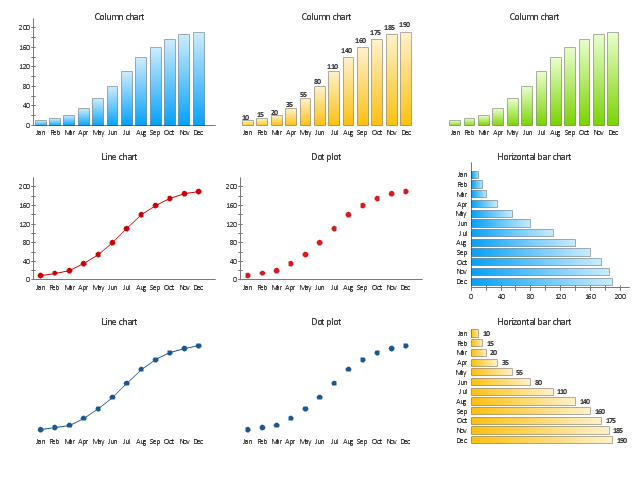# Exporting multiple graphs in same plot to PDF in R – TopBullets.comAs being a data scientist, plotting data is one of the first things we generally do. Without studying the behavior of the data we can’t or rather should not move ahead. There can be a lot of analysis which we can perform by plotting the graphs for example univariate, bivariate and residual plots. In my earlier blog, I wrote about how to plot two graphs in the same plot using par () function in R which is very useful when we do bivariate analysis and want to see the behavior of 2 variables across different time duration. Today I will write how to export the plots in PDF and in a tabular format. Generally exporting plots in any format (JPG, PDF) is an easier task but when you have say 50 graphs, you won’t want 50 pages, rather you will want 4 graphs in one page which will be very easier to read or interpret. Continue reading

# How to plot two graphs in the same plot in R and R ShinyWe as data analyst always have to do basic EDA or data manipulating before sorting the variable for developing any model. One of the first steps that we follow in EDA is bi-variate analysis. I will not go into details how or why we do bi-variate analysis, as you must know already and just want to learn how to plot 2 graphs in the same plot area in R. So let’s get started. I simply wrote an R code with comments to understand each step. Please check out the code below and comment if any step is not clear. Good luck. Continue readingHello Friends!